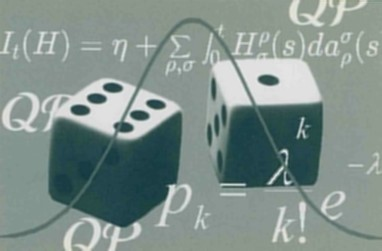# QP historyQuantum Probability is the mathematical theory arising from the rules for computing probabilities in Quantum Mechanics. J. von Neumann stated the fundamental axioms in the thirties, at the same time of Kolmogorov’s axioms of classical probability. His work, however, covered only some fundamental ideas of what could be called a “non-commutative measure theory”. After his pioneer researches, foundations of quantum mechanics were greatly enriched by new physical and mathematical ideas.

The systematic development of Quantum Probability started in the seventies when random variables, stochastic independence, stochastic processes, conditional expectations, stochastic calculus and Markov processes were studied in the framework of the probability theory arising from the von Neumann’s axioms and generalizations.

Quantum Probability nowadays is an established discipline with applications in probability theory, mathematical physics and functional analysis.